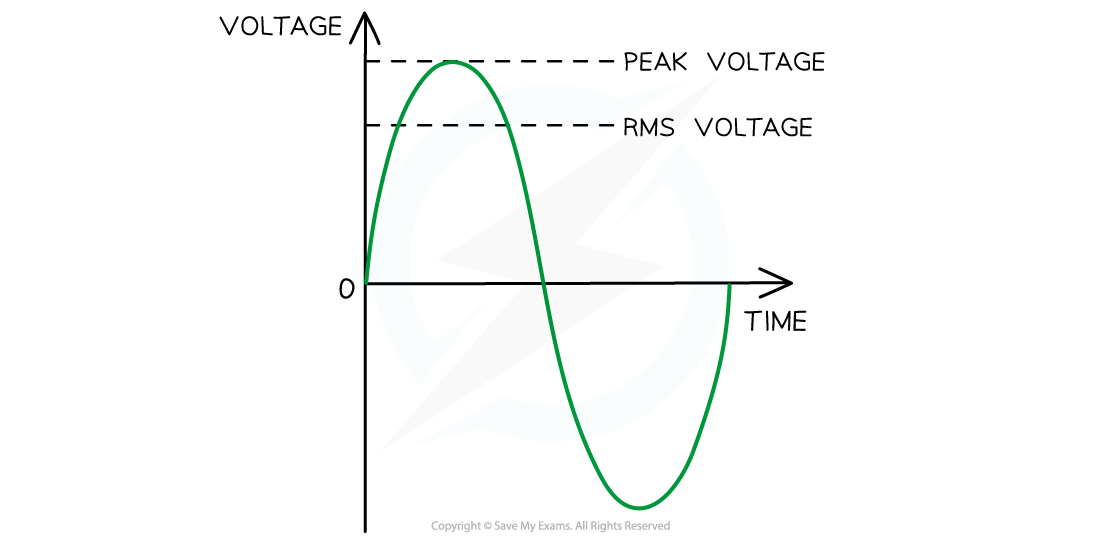# CIE A Level Physics复习笔记21.1.2 Root-Mean-Square Current & Voltage

### Root-Mean-Square Current & Voltage

• Root-mean-square (r.m.s) values of current, or voltage, are a useful way of comparing a.c current, or voltage, to its equivalent direct current, or voltage
• The r.m.s values represent the d.c current, or voltage, values that will produce the same heating effect, or power dissipation, as the alternating current, or voltage
• The r.m.s value of an alternating current is defined as:

The value of a constant current that produces the same power in a resistor as the alternating current

• The r.m.s current Ir.m.s is defined by the equation:• So, r.m.s current is equal to 0.707 × I0, which is about 70% of the peak current I0
• The r.m.s value of an alternating voltage is defined as:

The value of a constant voltage that produces the same power in a resistor as the alternating voltage

• The r.m.s voltage Vr.m.s is defined by the equation:• Where:
• I0 = peak current (A)
• V0 = peak voltage (V)
• The r.m.s value is therefore defined as:

The steady direct current, or voltage, that delivers the same average power in a resistor as the alternating current, or voltage

•  A resistive load is any electrical component with resistance eg. a lampVr.m.s and peak voltage. The r.m.s voltage is about 70% of the peak voltage

#### Worked Example

An alternating current is I is represented by the equation

I = 410 sin(100πt)

where I is measured in amperes and t is in seconds.For this alternating current, determine the r.m.s current.

Step 1: Write out the equation for r.m.s currentStep 2: Determine the peak voltage I0

• The alternating current equation is in the form: I = Isin(⍵t)
• Comparing this to I = 410 sin(100πt) means the peak current is I0 = 410 A

Step 3: Substitute into the Ir.m.s equation